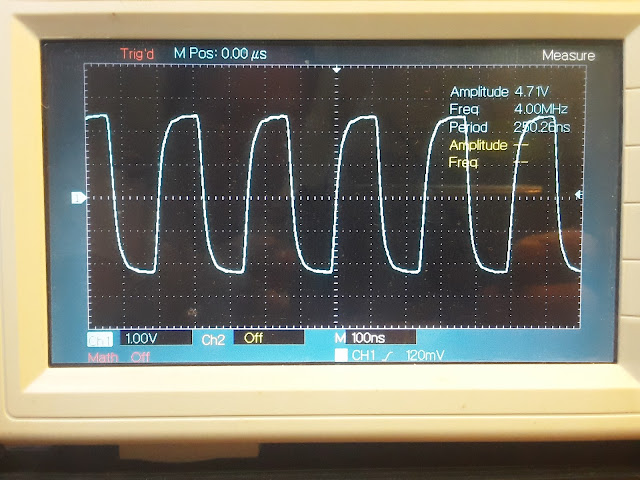# Arduino 8MHz Signal Generator with ISR

Here Arduino signal generator using ISR(Interrupt Service Routine) is demonstrated. In the previous tutorial Arduino 8MHz Variable Frequency Generator the interrupt service routine was not used but here we will use the interrupt service routine to generate signal. The Arduino Mega 2560 is used here but any other 8 bit Arduino board such as Arduino Uno, Arduino Nano etc can be used. One just has to make minor changes in the TCCR registers and change the output compare pin.

Here the CTC toggle mode is used to generate the adjustable frequency signal. The Timer 0 is used to generate the signal by loading OCR0A register with count value. By changing the count value we can change the frequency of the output signal. The output signal appears at the output compare pin OC0A which is pin 13 in Arduino Mega. An explanation with program code of how CTC mode works was presented in the tutorial Programming Arduino Mega in CTC mode and Arduino CTC mode Programming with Examples for using Arduino Uno. The frequency is adjusted using a 10KOhm potentiometer which is connected to one of the analog pin, here A0. As the potentiometer is rotated analog voltage received by the Arduino changes. The analog voltage is converted by the internal ADC(Analog to Digital Converter) to digital level in the range 0 to 1023. This digital level is converted to OCR0A acceptable range 0 to 255. The converted OCR0A level is loaded into the OCR0A. In this way the signal changes from the potentiometer changes the OCR0A value and hence the frequency of the output square wave.

The following shows the circuit diagram of the Arduino signal generator is shown below.

The following shows how the Arduino is connected on a breadboard.

The following is the Arduino program code for the signal generator.


const int N=1;
const float Fcpu = 16000000;
int anaValue;

void setup() {
//set OC0A as output
pinMode(13, OUTPUT);
//set up serial
Serial.begin(9600);

// reset timer 0 control registers
TCCR0B = 0;
TCCR0A = 0;

// Load 255 to generate 31.25 kHz sq.wave
OCR0A = 255;
// Toggle OC0A on compare match, WGM 2 (CTC)
TCCR0A |= (1 << COM0A0) | (1 << WGM01);
// Start the timer, no prescalar
TCCR0B |= (1 << CS00);
//Setup Interrupt
TIMSK0 |= (1<<OCIE0A);
//set global interrupt
sei();
}

void loop() {
float Fw = (Fcpu)/((1+OCR0A)*2*N);

Serial.print("OCR0A: ");
Serial.print(OCR0A);
Serial.println();
Serial.print("Freq.: ");
Serial.print(Fw);
Serial.print("Hz");
Serial.println();

}

ISR(TIMER0_COMPA_vect){
OCR0A = map(anaValue, 0,1023, 0, 255);
}

In the above square wave generator code, we have configured the Timer 0 in CTC toggle mode with pre scalar value of 1. This is done by configuring the TCCR0A and TCCR0B register. The compare interrupt bit OCIE0A for compare unit A of Timer 0 which is located in the TIMSK0 register is enabled. In the main loop we read in the analog value and store the value in the variable anaValue. When the count is reached the ISR(Interrupt Service Routine) is called and in the service routine we map the ADC value to OCR0A range and load the converted value into the OCR0A register.

The following picture shows the 31.25KHz, 4MHz and 8MHz square wave signal.

8MHz signal:

4MHz signal31.25KHz signal:

Watch the following video on how the Arduino Signal Generator works.

Also if you want DIY home electronics testing tools then see the following tutorials.